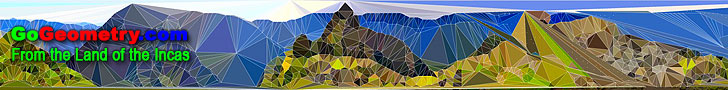# Recent additions (Page 27), Online LearningGo Geometry from the Land of the Incas - Recent Additions (Page 27) Problem 533. Euclid's Elements, Book XIII, Proposition 10. One page visual illustration. Brahmagupta Theorem and Problems - Index Brahmagupta (598–668) was an Indian mathematician and astronomer who discovered a neat formula for the area of a cyclic quadrilateral. Problem 532. Triangle, Circumcircle, Equal Angles, Perpendicular, Area. Problem 531. Triangle, Angle Bisector, Midpoint, Parallel, Perimeter, Semiperimeter. Semiperimeter, Perimeter Index. Theorems and Problems. Geometry Problems 241 - 250. Triangle, Quadrilateral, Congruence, Area, Parallelogram, Square, Diagonal, Center, Napoleon, Median, Midpoint. Problem 530. Cyclic Quadrilateral, Diagonal, Diameter, Perpendicular, Congruence. Problem 529. Right Trapezoid, Circle, Diameter. Geometry Problems 231 - 240. Triangle, Quadrilateral, Square, Parallelogram, Trapezoid, Altitude, Distances, Midpoint, Perpendicular, Transversal. Problem 528. Triangle, Medians, Perpendicular, Measurement. Go to Page: Previous | 1 | 2 | 3 | 4 | 5 | 6 | 7 | 8 | 9 | 10 | 11 | 12 | 13 | 14 | 15 | 16 | 17 | 18 | 19 | 20 | 21 | 22 | 23 | 24 | 25 | 26 | 27 | 28 | 29 | 30 | 31 | 32 | 33 | 34 | 35 | 36 | 37 | 38 | 39 | 40 | 41 | 42 | 43 | 44 | 45 | 46 | 47 | 48 | 49 | 50 | Next

 Home | GoGeometry Education | Geometry | Search | Email